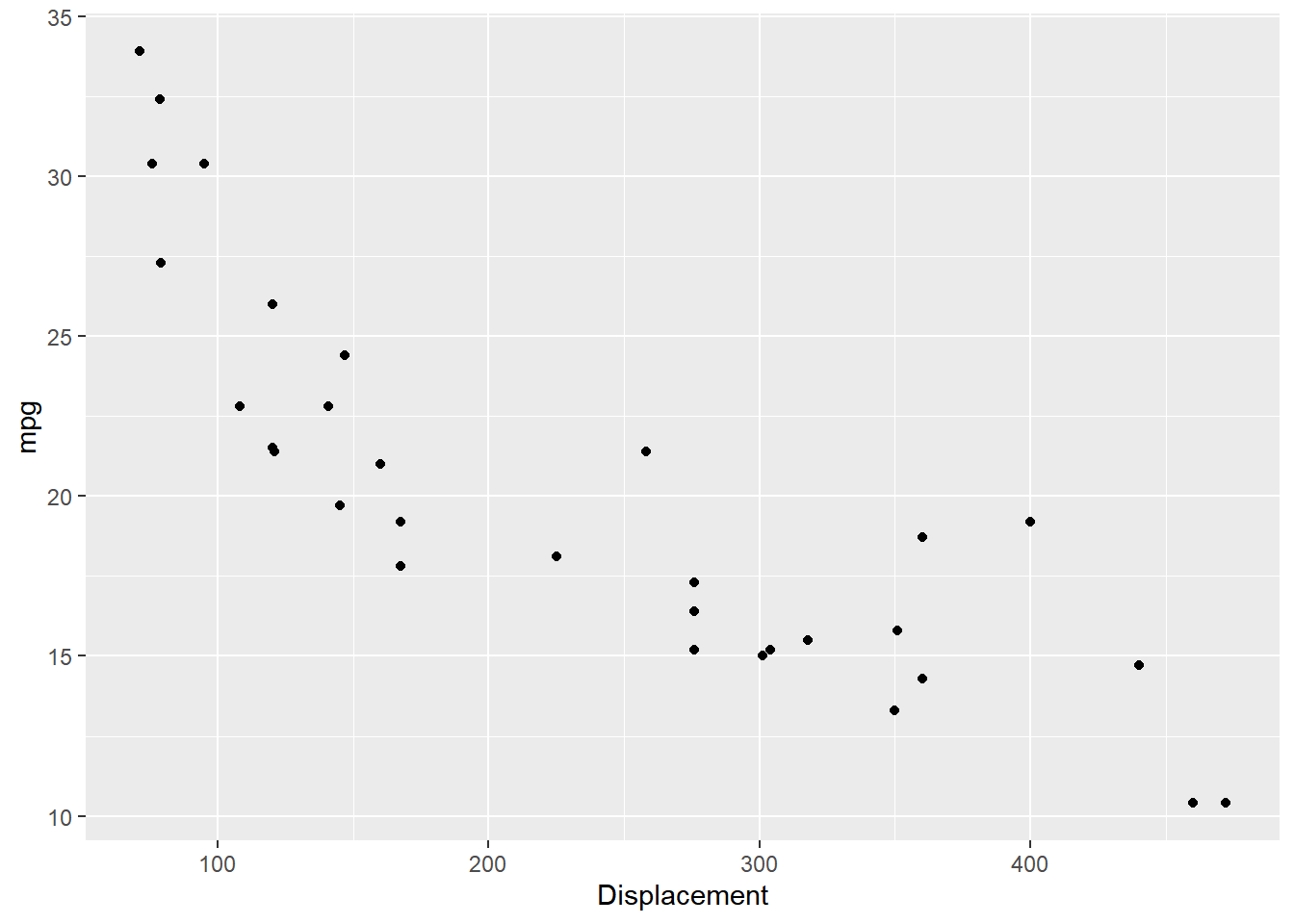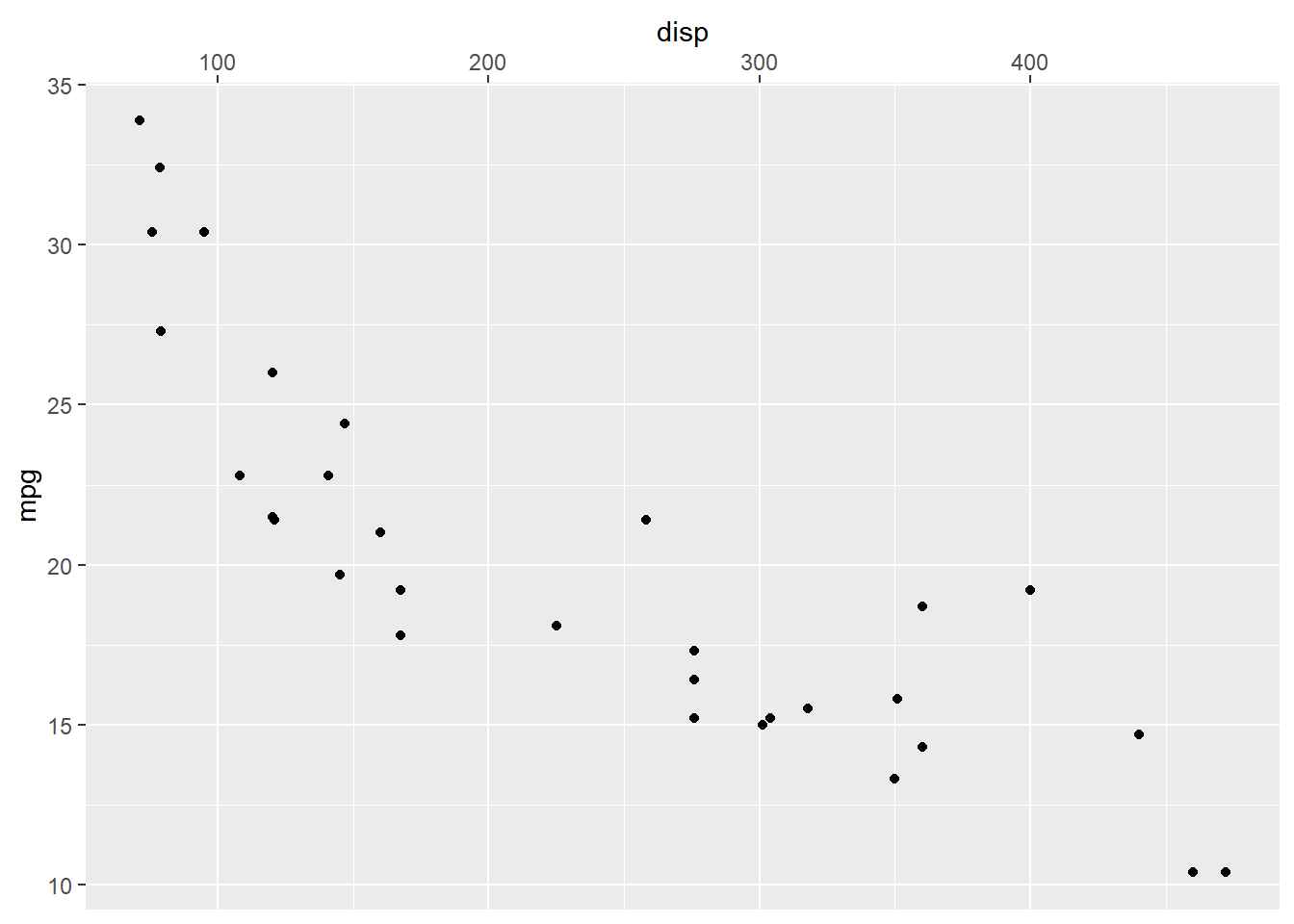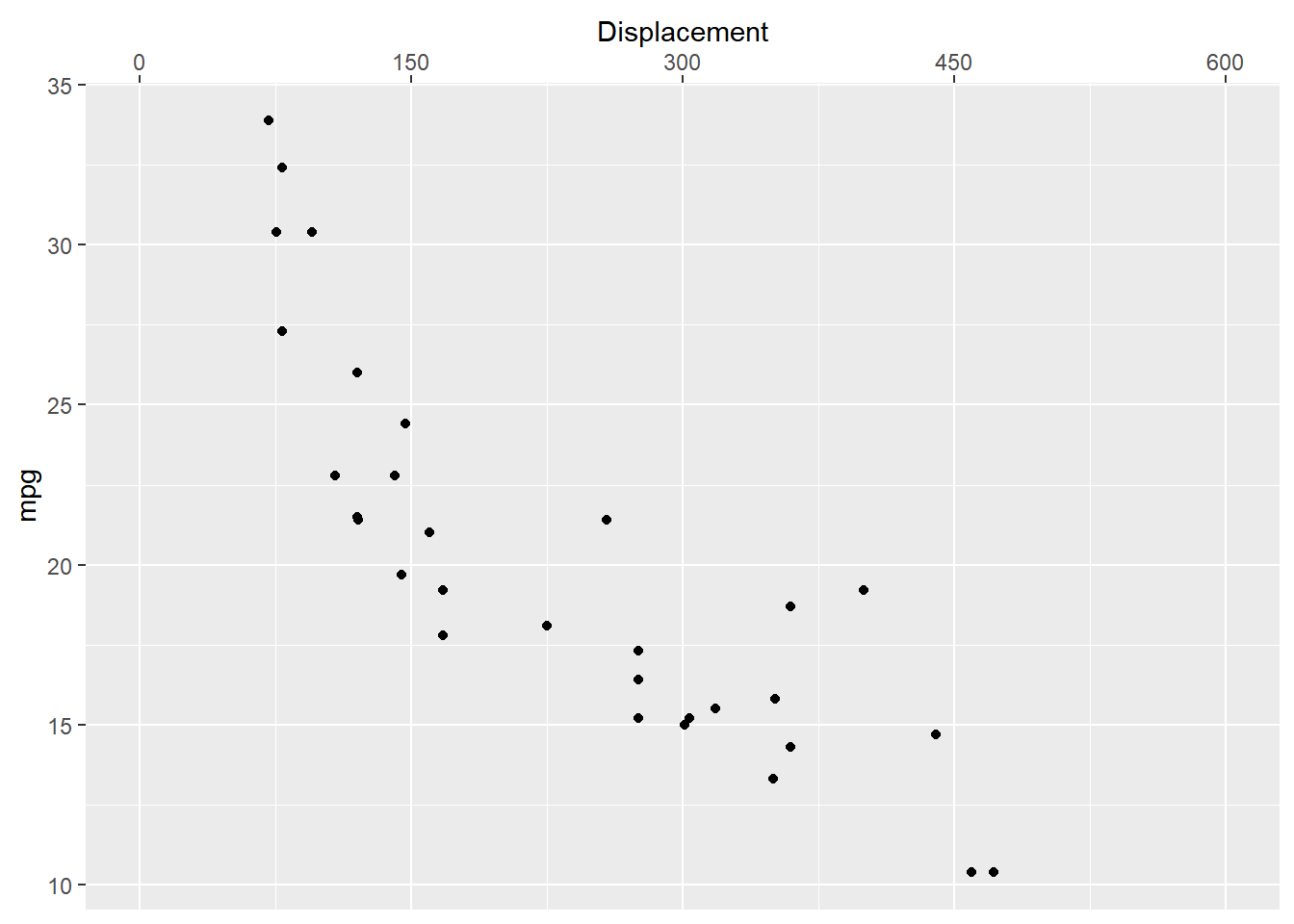Want to share your content on R-bloggers? click here if you have a blog, or here if you don't.

### Introduction

This is the twelfth post in the series Elegant Data Visualization with
ggplot2
. In the previous post, we learnt to build histograms. Now that we
have learnt to build different plots, let us look at different ways to modify
the axis. Along the way, we will also explore the `scale_*()` family of functions.

Modify X and Y axis

• title
• labels
• limits
• breaks
• position

In this module, we will learn how to modify the X and Y axis using the
following functions:

• Continuous Axis
• `scale_x_continuous()`
• `scale_y_continuous()`
• Discrete Axis
• `scale_x_discrete()`
• `scale_y_discrete()`

### Libraries, Code & Data

We will use the following libraries in this post:

All the data sets used in this post can be found here

### Continuous Axis

If the X and Y axis represent continuous data, we can use
`scale_x_continuous()` and `scale_y_continuous()` to modify the axis. They take
the following arguments:

• name
• limits
• breaks
• labels
• position

Let us continue with the scatter plot we have used in previous posts.

```ggplot(mtcars) +
geom_point(aes(disp, mpg))```The `name` argument is used to modify the X axis label. In the below example,
we change the X axis label to `'Displacement'`. In previous posts, we
have used `xlab()` to work with the X axis label.

```ggplot(mtcars) +
geom_point(aes(disp, mpg)) +
scale_x_continuous(name = "Displacement")```To modify the range, use the `limits` argument. It takes a vector of length
2 i.e. 2 values, the lower and upper limit of the range. It is an alternative
for `xlim()`.

```ggplot(mtcars) +
geom_point(aes(disp, mpg)) +
scale_x_continuous(limits = c(0, 600))```In the above plot, the ticks on the X axis appear at `0`, `200`, `400` and
`600`. Let us say we want the ticks to appear more closer i.e. the difference
between the tick should be reduced by `50`. The `breaks` argument will allow
us to specify where the ticks appear. It takes a numeric vector equal to the
length of the number of ticks.

```ggplot(mtcars) +
geom_point(aes(disp, mpg)) +
scale_x_continuous(breaks = c(150, 300, 450))```We can change the tick labels using the `labels` argument. In the below
example, we use words instead of numbers. When adding labels, we need to
ensure that the length of the `breaks` and `labels` are same.

```ggplot(mtcars) +
geom_point(aes(disp, mpg)) +
scale_x_continuous(breaks = c(150, 300, 450),
labels = c('One Hundred Fifty', 'Three Hundred', 'Four Hundred Fifity'))```The position of the axes can be changed using the `position` argument. In the
below example, we can move the axes to the top of the plot by supplying the
value `'top'`.

```ggplot(mtcars) +
geom_point(aes(disp, mpg)) +
scale_x_continuous(position = 'top')```### Putting it all together..

```ggplot(mtcars) + geom_point(aes(disp, mpg)) +
scale_x_continuous(name = "Displacement", limits = c(0, 600),
breaks = c(0, 150, 300, 450, 600), position = 'top',
labels = c('0', '150', '300', '450', '600'))```### Y Axis – Continuous

```ggplot(mtcars) + geom_point(aes(disp, mpg)) +
scale_y_continuous(name = "Miles Per Gallon", limits = c(0, 45),
breaks = c(0, 15, 30, 45), position = 'right',
labels = c('0', '15', '30', '45'))```### Discrete Axis

If the X and Y axis represent discrete or categorical data, `scale_x_discrete()`
and `scale_y_discrete()` can be used to modify them. They take the following arguments:

• name
• labels
• breaks
• position

The above options serve the same purpose as in the case of continuous scales.

#### Axis Label

```ggplot(mtcars) +
geom_bar(aes(factor(cyl))) +
scale_x_discrete(name = "Number of Cylinders")```#### Axis Tick Labels

```ggplot(mtcars) +
geom_bar(aes(factor(cyl))) +
scale_x_discrete(labels = c("4" = "Four", "6" = "Six", "8" = "Eight"))```#### Axis Breaks

```ggplot(mtcars) +
geom_bar(aes(factor(cyl))) +
scale_x_discrete(breaks = c("4", "6", "8"))```#### Axis Position

```ggplot(mtcars) +
geom_bar(aes(factor(cyl))) +
scale_x_discrete(position = 'bottom')```#### Putting it all together…

```ggplot(mtcars) + geom_bar(aes(factor(cyl))) +
scale_x_discrete(name = "Number of Cylinders",
labels = c("4" = "Four", "6" = "Six", "8" = "Eight"),
breaks = c("4", "6", "8"), position = "bottom")```### Summary

In this post, we learnt to modify

• title
• labels
• limits
• breaks
• position

### Up Next..

In the next post, we will learn to modify the legend when `color` is mapped to a variable.